06 325 761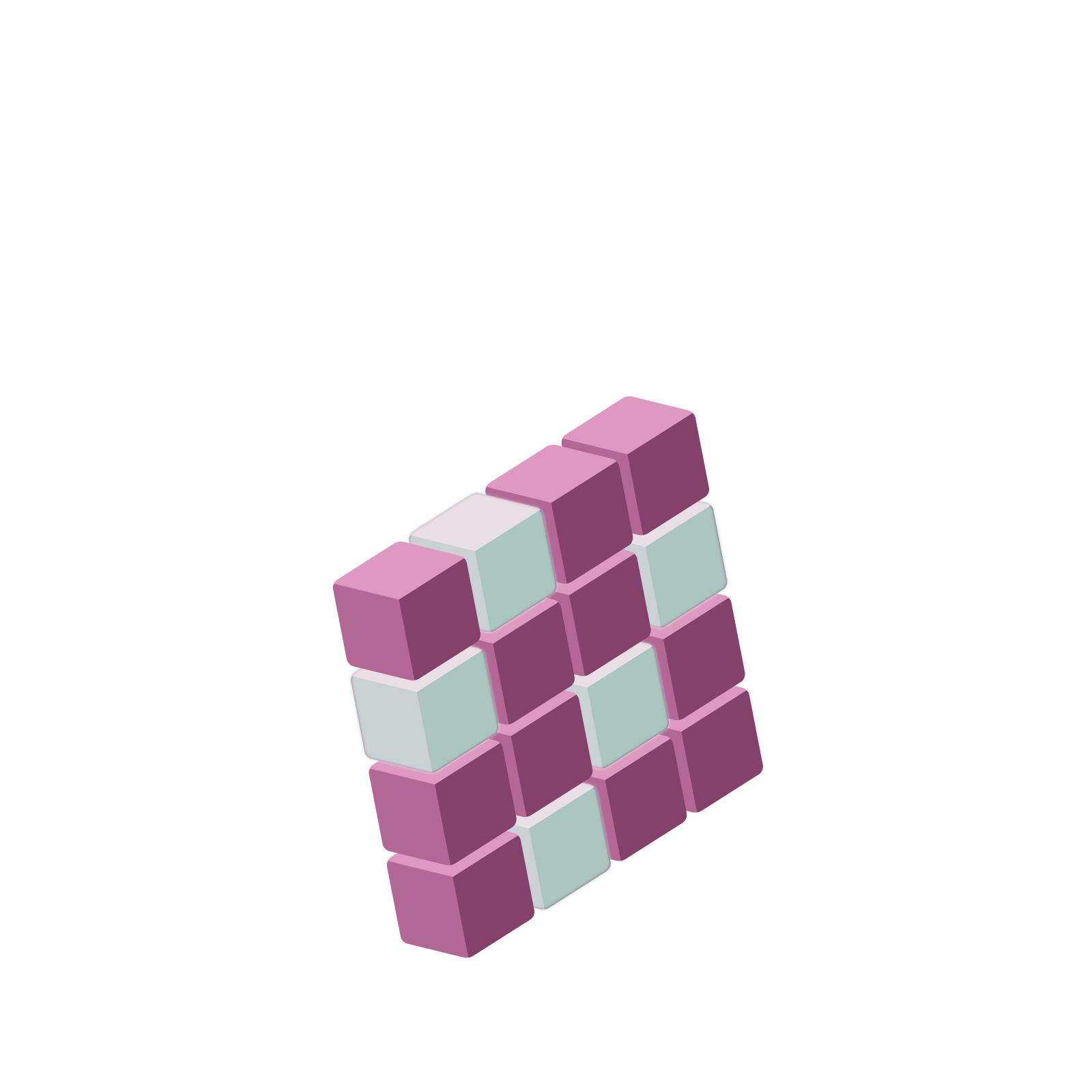# Station  10AA Troubling Number for our Usual MathematicsHere’s a deep age-old question regularly asked by young math scholars across the globe.

Is $0.9999\ldots$ equal to one or is it not?

This is a quantity with infinitely many $9$s listed to the right of the decimal point.Well, let’s be honest, that’s a dubious issue at the outset: we didn’t actually list infinitely many $9$s to the right. We humans never can. We might have the patience to write down twenty $9$s, or two hundred $9$s, or we might hire a team of two thousand people to work for twenty years to list out two billion of those $9$s , but we humans will never see, actually see, an infinite number of $9$s written to the right of the decimal point. We have to cheat and write an ellipsis, “$\ldots$”, to say “imagine this going on forever.”

So right away we should realise that the question asked is one for the mind. It is really asking:

If we were somehow God-like and could list an infinite string of $9$s to the right of the decimal point, would the quantity that results be equal to $1$, or not?

And one might decide that since we humans are not God-like the question is meaningless—what does “the quantity that results” mean?—and so there is no point to even giving the question consideration.

That’s a valid stance to take.

But, nonetheless, this question keeps coming up. We are teased by it. And it seems we do want to try to play with it and make sense of it. Somehow it feels as though we humans, although not able to actually see and write down the infinite, can somehow envision the infinite, that we’re just a small step away from having a grasp of it.

Each approximation we humans write for $0.9999\ldots$ is close to $1$, not equal to $1$, in fact, just shy of $1$ each time.

$0.9$ is less than $1$.

$0.99$ is less than $1$.

$0.99999$ is less than $1$.

$0.999999999999$ is less than $1$.

$0.9999999999999999999999999999999999999999999$ is less than $1$.

And some people use this to argue that $0.9999\ldots$ too must be smaller than $1$, and so not equal to $1$.

But let’s think a little more deeply about these numbers $0.9$, $0.99$, $0.999$, …. They’re are sequence of numbers marching rightward on the number line, heading to the number $0.9999\ldots$, which might or might not be $1$.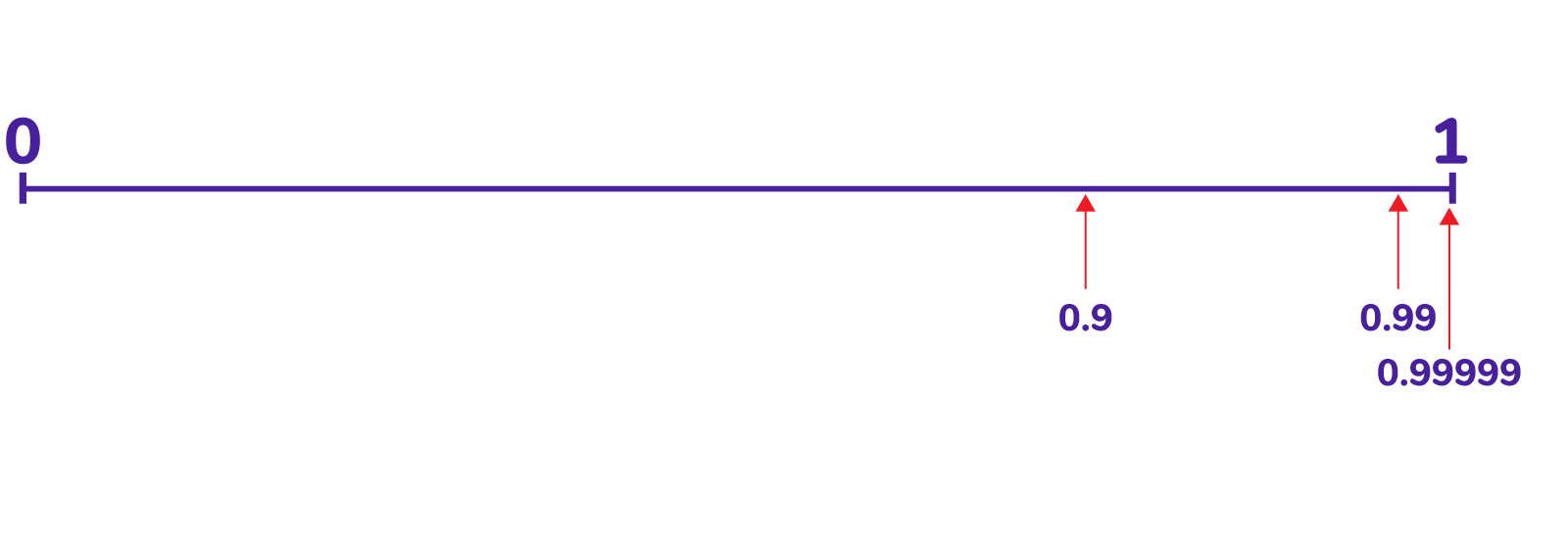At the same time, we see the more $9$s we list after the decimal point, the closer the numbers are to the number $1$. For instance, $0.99$ is one-hundredth of a unit away from $1$, and $0.999999$ is one-millionth of a unit away from $1$.

So in fact the numbers $0.9$, $0.99$, $0.999$, … steadily march rightward on the number line eventually entering any amount of space you might specify sitting to the left side of $1$. (Want a number within a distance of $\frac{1}{337}$ from $1$? $0.9999$ will do!)

So could the quantity $0.9999\ldots$ be a number sitting just shy of the number $1$ and not be $1$? Could there be any empty space between it and $1$?

No! We just argued that the sequence of $0.9$, $0.99$, $0.999$, … will march into any space we specify to the left of $1$! Thus we could conclude

There can be no space between $0.9999\ldots$ and $1$ on the number line, and so $0.9999\ldots$ must actually be $1$.

Well … not quite!

There is another possibility to rule out.

Could $0.9999\ldots$ instead be quantity sitting just to the right of $1$ on the number line?

This feels unlikely since all the numbers $0.9$, $0.99$, $0.999$, … sit to the left of $1$ with $1$ being an upper barrier to them all.

But if we feel uneasy about a geometric argument, we can always turn to an algebraic one.

## AN ALGEBRAIC ARGUMENT

If you choose to believe that $0.9999\ldots$ is a valid arithmetical quantity (that might or might not be $1$), then you probably would also choose to believe that it obeys all the usual rules of arithmetic. If so, then we can conduct a lovely swift algebra argument so show that, because of these beliefs, the quantity $0.9999\ldots$ does indeed equal $1$. The argument goes as follows.

STEP 1: Give the quantity a name.

We’ll call it $F$ for Fredericka:

$F = 0.9999\ldots$.

STEP 2: Multiply by ten

We obtain

$10F = 9.9999\ldots$.

STEP 3: Subtract

Notice $10F$ and $F$ differ only by $9$ in the ones place and so $10F - F$ is $9$. That is, we have

$9F=9$

giving

$F=1$. .

The mathematics thus establish that $0.9999\ldots = 1$.

Lovely!

But let me be clear on what we have established here.

IF you choose to believe that $0.9999\ldots$ is a meaningful quantity in USUAL MATHEMATICS, then you must conclude that it equals $1$.

I say this because this algebraic argument can lead to philosophical woes, as we’ll see in the next lesson.

## FINAL TOUGHT

Here’s a picture of $0.9999\ldots$ in a $1 \leftarrow 10$ machine with decimals.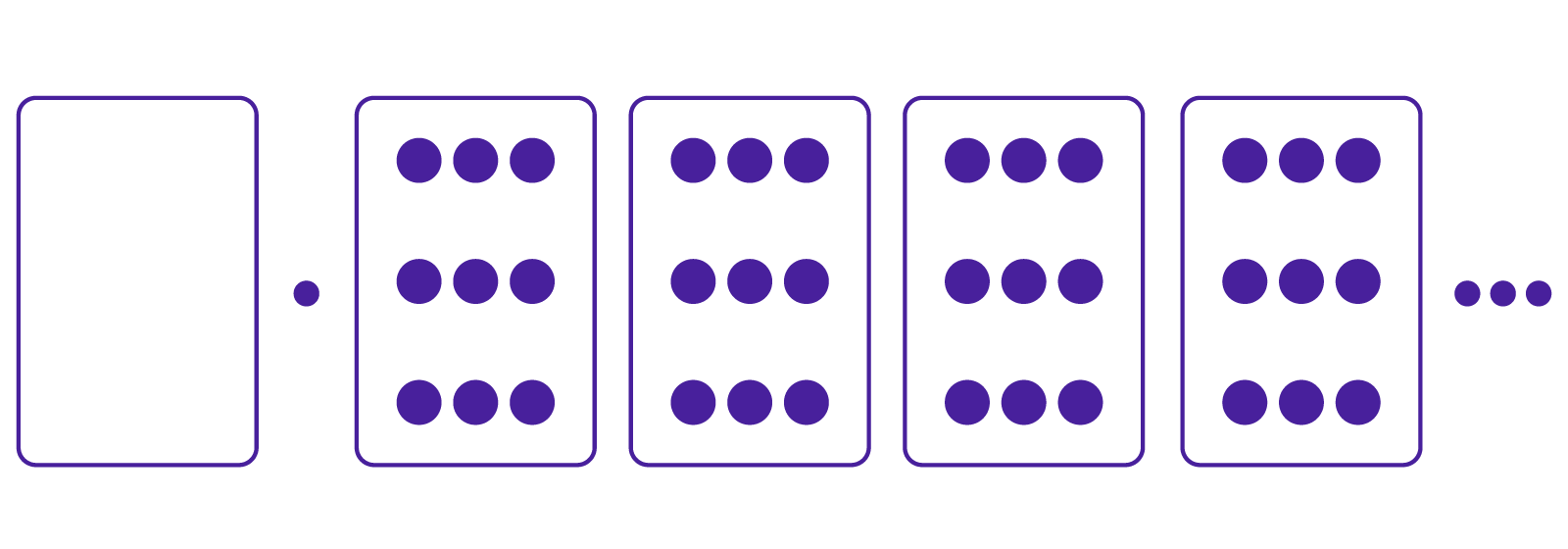Place a dot and an antidot in the first box. This doesn’t change the number.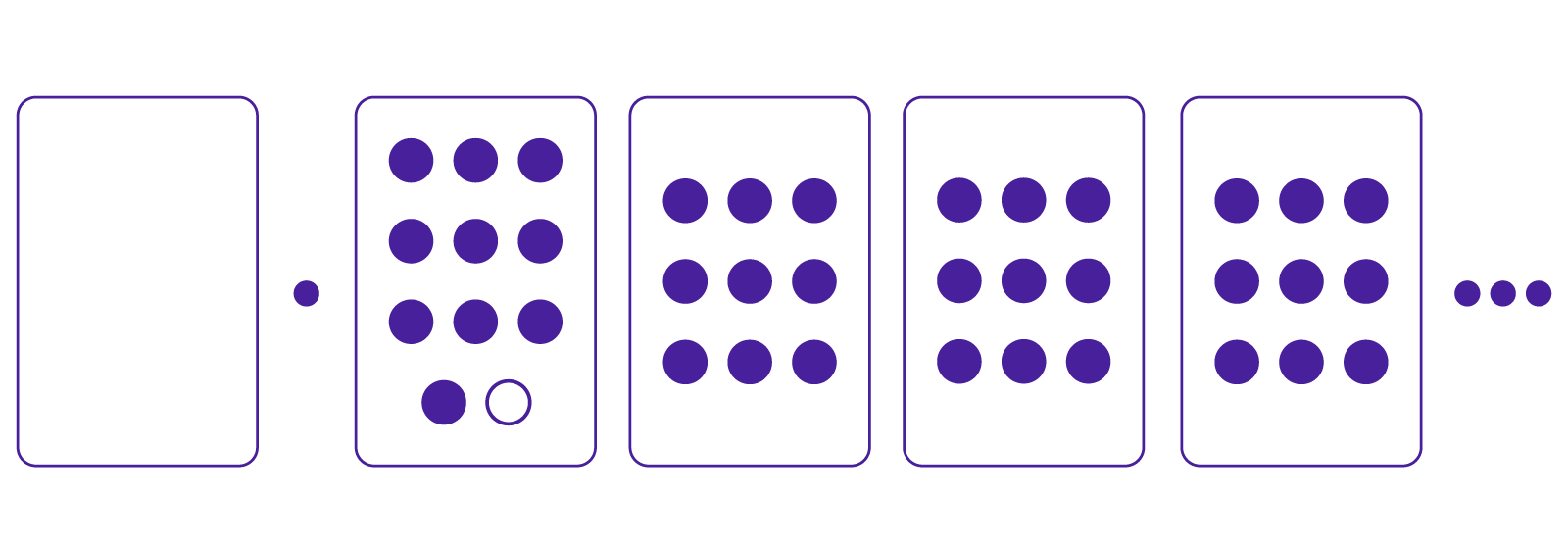But now we can perform an explosion.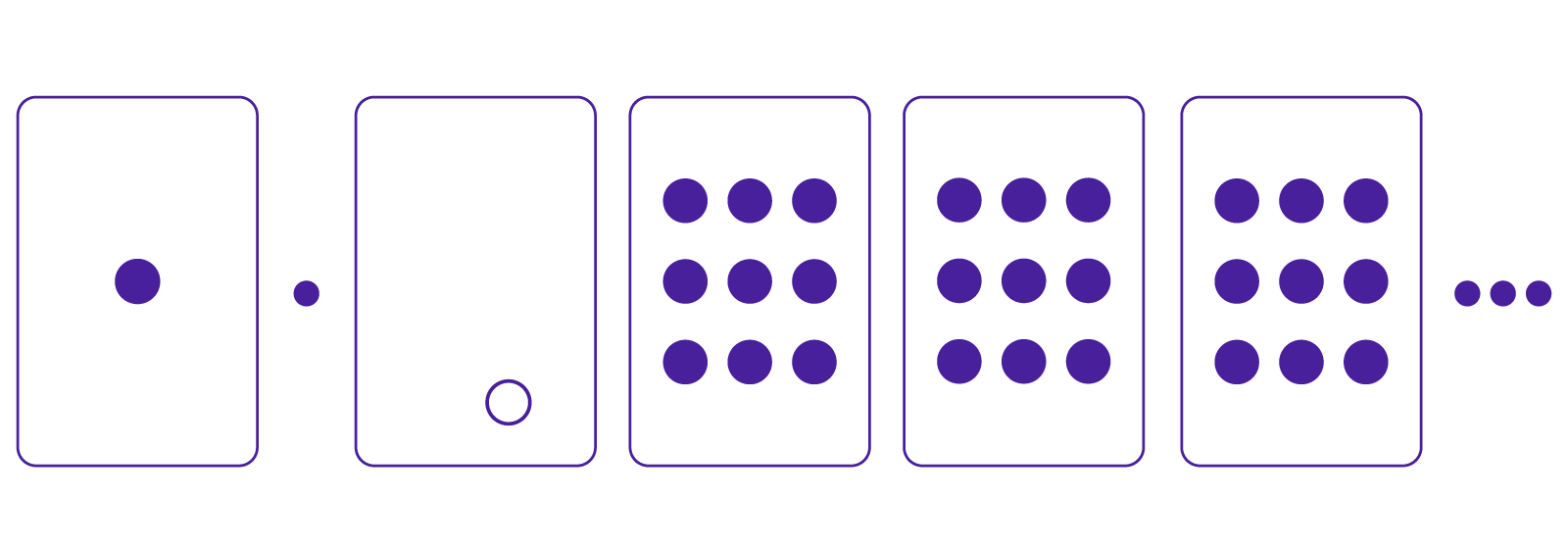The number in this picture which looks like $1.-1|9|9|9\ldots$ is still $0.9999\ldots$.

Now add another dot-antidot pair and perform another explosion. There is now also an annihilation.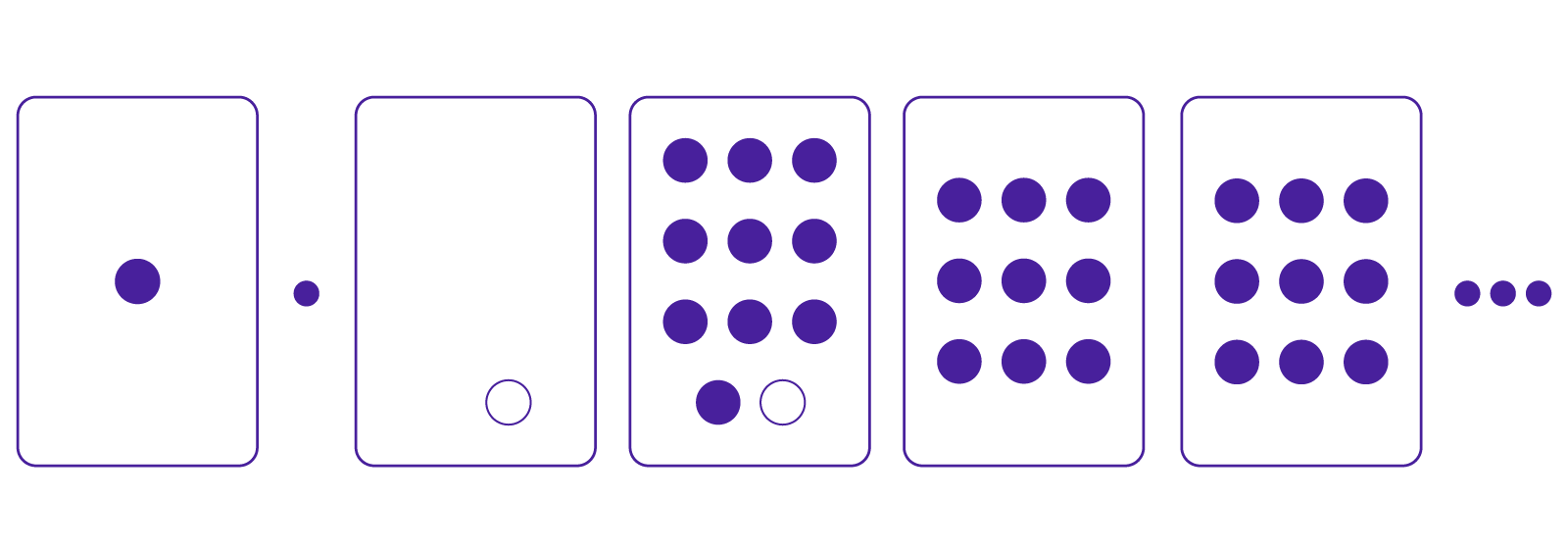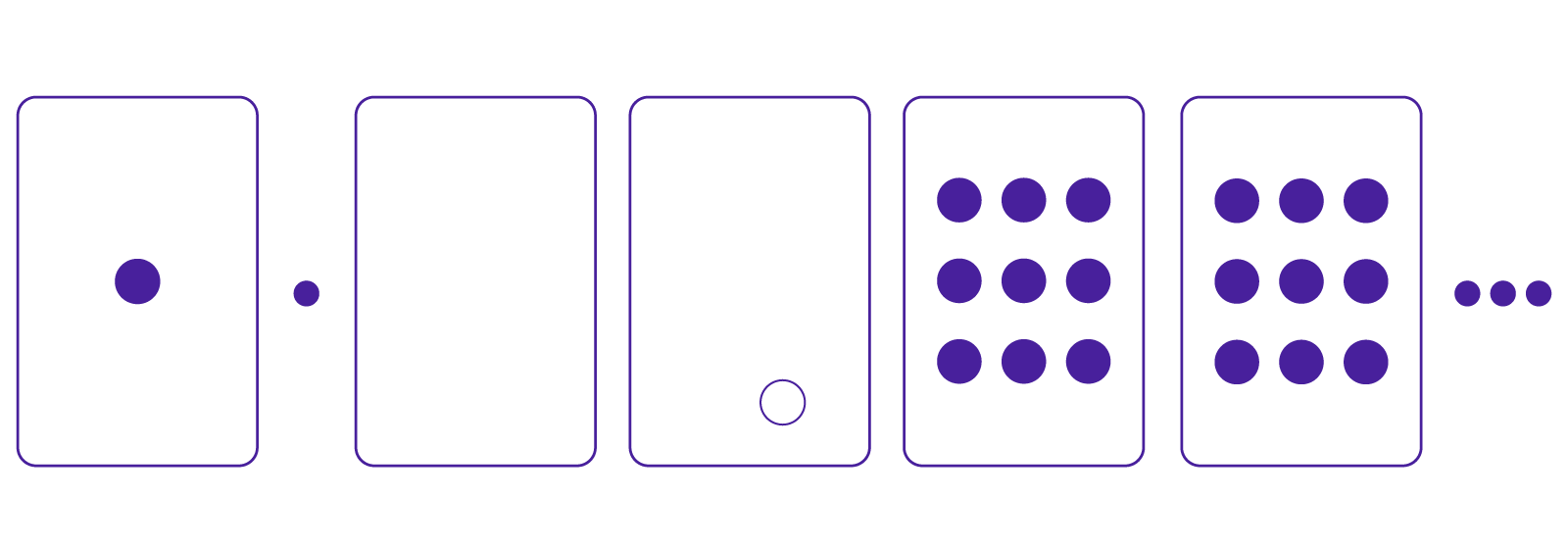Now we have $1.0|-1|9|9\ldots$ is the same as $0.9999\ldots$.

And do this again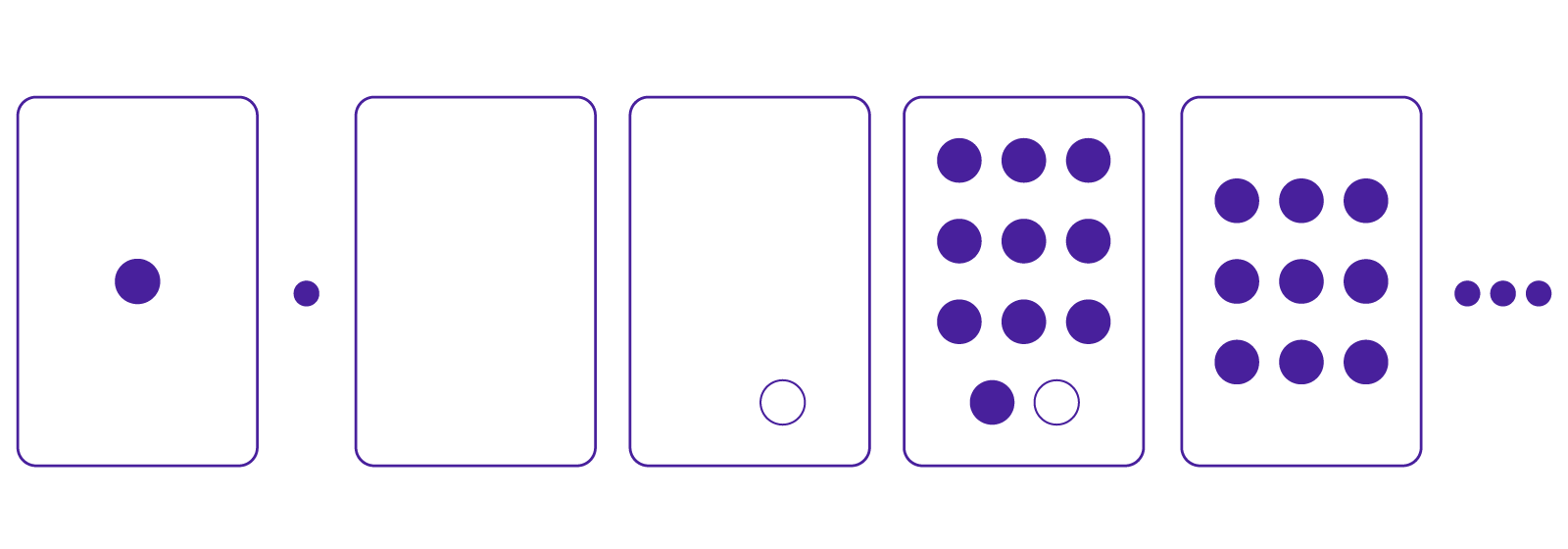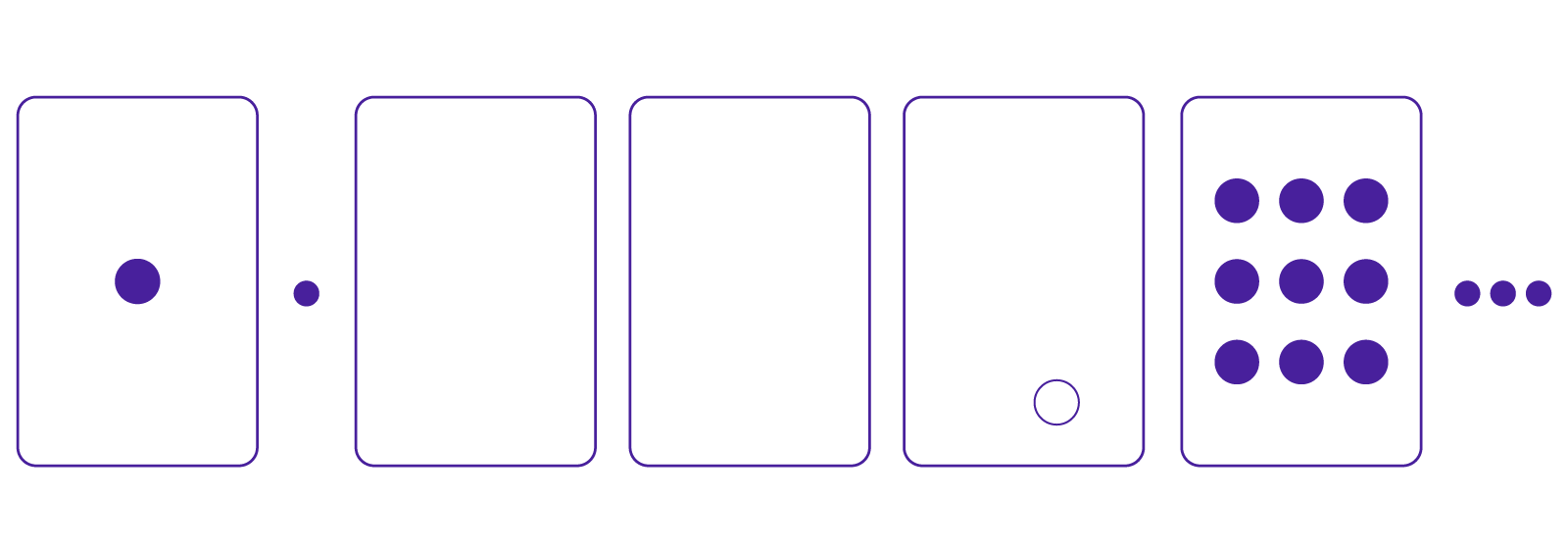and again, and again, and again, forever.

It looks like we are actually showing that $0.9999\ldots$ is the same as $1.0000\ldots$.